§15.18. Equations

Forming totals is all very interesting in its way, but it's book-keeping rather than physics. As a glance at any school science textbook shows, the way to apply physics is to work out an unknown quantity - say, the time taken for a dropped ball to hit the ground - by combining known quantities into an equation - the height it is dropped from, and the strength of gravity.

It's a convention centuries old now that textbooks and research papers never describe these equations in running text. Even for simple formulae, we like to write "F=ma", not "let the force be the mass times the acceleration". And the standard way to print this is to break off and display an equation, not to squeeze it into the text as if it were ordinary verbiage. Just as Inform's Tables imitate those in printed books (see the next chapter), so its Equations do.

In this section, we'll use a combination of three equations to work out how soon and how hard an object pushed off a table will hit the floor. First, we'll include Metric Units, to define all of the kinds of value and notations we need.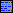Include Metric Units by Graham Nelson.

Now we'll give everything a mass (Metric Units likes to talk about mass instead of weight, but on Earth it's the same thing) and also set up a typical strength for gravity - it's a little less at the poles, a little more at the equator, but this is the conventional approximate value to use.

The acceleration due to gravity is an acceleration that varies. The acceleration due to gravity is usually 9.807 m/ss. A thing has a mass. The mass of a thing is usually 10g.

To a Renaissance scientist, typically living in a walled European town, a cannon ball was a familiar thing, and it often featured in imaginary experiments:

Laboratory is a room. The cannon ball is in the Laboratory. "A cannon ball perches delicately on a lab bench." The mass of the cannon ball is 2kg.

And now we're ready for the three equations. These will all have names, but we could just as easily have numbered them, calling them (say) "Equation 1", "Equation 2" and "Equation 3".

Equation - Newton's Second Law
F=ma
where F is a force, m is a mass, a is an acceleration.

Equation - Principle of Conservation of Energy
mgh = mv^2/2
where m is a mass, h is a length, v is a velocity, and g is the acceleration due to gravity.

Equation - Galilean Equation for a Falling Body
v = gt
where g is the acceleration due to gravity, v is a velocity, and t is an elapsed time.

An equation has to take the form of one formula equals another, where each formula is made up from symbols defined afterwards. The symbols can be defined as definite values (as "g" is defined in the Galilean Equation), or just by telling Inform their kinds of value (as "v" and "t" are defined).

Equations are read using standard mathematical conventions. So "x + yz" means that we multiply y and z, then add that to x; "ab/cd" divides the product of a and b by the product of c and d. Multiplication signs can be omitted, just as science books normally do (though we can always write them if we want to, using the asterisk *, as usual in computing). The need for brackets is minimised, with any luck, but we can use them if we need to: "x(y+ab)" is legal, for instance.

One difference between Inform's conventions and mathematical ones, though, is that Inform generally ignores upper-versus-lower-case when reading variable names, so it wouldn't be a good idea to write "F = gMm/r^2" and expect "M" and "m" to be different from each other.

Here is the calculation:

Instead of pushing the cannon ball:
let the falling body be the cannon ball;
let m be the mass of the falling body;
let h be 1.2m;
let F be given by Newton's Second Law where a is the acceleration due to gravity;
let v be given by the Principle of Conservation of Energy;
let t be given by the Galilean Equation for a Falling Body;
say "You push [the falling body] off the bench, at a height of [h], and, subject to a downward force of [F], it falls. [t to the nearest 0.01s] later, this mass of [m] hits the floor at [v].";
now the falling body is in the location.

And the result is:

You push the cannon ball off the bench, at a height of 1.2m, and, subject to a downward force of 19.614N, it falls. 0.49s later, this mass of 2.0kg hits the floor at 4.85147 m/s.

Not all that fast-moving - it's only about 10 mph, ten times slower than one fired by a Renaissance cannon - but half a second wouldn't give you long to get your foot out of the way.

How was that done? The crucial lines are the ones in the form "let X be given by E...", which is a new form of "let".

let (a name not so far used) be given by (equation name)

or:

let (a temporary named value) be given by (equation name)

This phrase creates a new temporary variable, starting it with the value found by solving the given equation. The variable lasts only for the present block of phrases, which certainly means that it lasts only for the current rule. Example:

let F be given by Newton's Second Law where a is the acceleration due to gravity;

There is also a more compact syntax, giving the equation explicitly:

let KE be given by KE = mv^2/2 where KE is an energy;

When we solve with "let", then, all of the other symbols should either already have values (because they exist as "let" values already made) or else be specified in the line. For instance,

let F be given by Newton's Second Law where a is the acceleration due to gravity;

is allowed because "F" is one of the symbols in "F = ma"; of the other two symbols, we have a "let" variable called "m" already - it's the mass of the cannon ball - and we declare exactly what "a" is.

The next calculation is more interesting:

let v be given by the Principle of Conservation of Energy;

Since the equation here is "mgh = mv^2/2", Inform has to do some algebra to work out "v" in terms of the other unknowns - it's the square root of 2gh, but we don't need to work that out. Inform can't always solve implicit equations - for instance, it can't deduce "m" from this equation - but it's correct on all the easy cases which occur in basic physics, and that enables us to write equations in their most natural form, which is easier to read and understand.

The advantage of setting out an equation formally is that it can be used in many places - we could use Newton's Second Law again for something quite different, for example. But it's a little cumbersome for something simple which we only need once, so this is neater:

let KE be given by KE = mv^2/2 where KE is an energy;

Here the equation is written out explicitly instead of being named, but otherwise everything works in the same way.

Equations can also contain many of our standard functions, which are written for this purpose with their standard mathematical abbreviations. For example:

let x be given by sin x = 1 where x is a real number;

works out x as pi divided by 4, which is to say, 90 degrees. The Phrasebook entries on the mathematical functions give their abbreviations, but here they all are as a list:

abs, root, ceiling, floor, int, log, exp, sin, cos, tan, arcsin, arccos, arctan, sinh, cosh, tanh, arcsinh, arccosh, arctanh

As an example, here's the definition of arcsinh given in the Standard Rules:

To decide which real number is the hyperbolic arcsine of (R - a real number):
let x be given by x = log(R + root(R^2 + 1)) where x is a real number;
decide on x.

Something to be a little cautious of: brackets are used in equations to group terms together, and do not mean function application, as they would in a C-like programming language. For example, "sin(1+x)/2" takes the sine of "(1+x)/2": if we want to halve the sine of "1+x", we have to write "(sin(1+x))/2".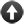Start of Chapter 15: Numbers and Equations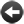Back to §15.17. Totals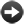Onward to §15.19. Arithmetic with units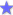ExampleWidget Enterprises Allowing the player to set a price for a widget on sale, then determining the resulting sales based on consumer demand, and the resulting profit and loss.